# The Fast Lifting Wavelet Transform

## Disclaimer

This tutorial is aimed at the engineer, not the mathematician. This does not mean that there will be no mathematics; it just means that there will be no proofs in the text. In my humble opinion, mathematical papers are completely unreadable because of the proofs that clutter the text. For proofs the reader is pointed to suitable references. The equations presented are there to illustrate and to clarify things, I hope. It should not be necessary to understand all the equations in order to understand the theory. However, to understand this tutorial, a mathematical background on an engineering level is required. Also some knowledge of signal processing theory might come in handy.

The information presented in this tutorial is believed to be correct. However, no responsibility whatsoever will be accepted for any damage whatsoever due to errors or misleading statements or whatsoever in this tutorial. Should there be anything incorrect, incomplete or not clear in this text, please let me know so that I can improve this tutorial.

## 1. Introduction

This tutorial is a sequel to the wavelet tutorial, which will fill in the blank spots on the wavelet transform map, add some detail and even explore the area outside it. We start with taking a closer look at the scaling and wavelet filters in general, what they should look like, what their constraints are and how they can be used in the inverse wavelet transform. Then we will do some algebra and develop a general framework to design filters for every possible wavelet transform. This framework was introduced by Sweldens [Swe96a] and is known as the lifting scheme or simply lifting. Using the lifting scheme we will in the end arrive at a universal discrete wavelet transform which yields only integer wavelet- and scaling coefficients instead of the usual floating point coefficients. In order to clarify the theory in this tutorial a detailed example will be presented.

In this tutorial we will go into some more detail compared to the wavelet transform tutorial, since the lifting scheme is a quite recent development and especially integer lifting [Cal96], [Uyt97b] and multi-dimensional lifting [Kov97], [Uyt97a] are not (yet) widely known. This tutorial is mainly based on [Dau97], [Cal96], [Swe96a], [Swe96b], [Cla97], [Uyt97b] and [Uyt97c].

Before we start a quick note on notation. In order to be compatible with existing lifting literature this tutorial will try to use the same symbols. However, using tildes proved to be rather difficult, so they are replaced by a hat symbol. This means that the analyzing filters are denoted asand, i.e. with a hat, while the synthesizing filters are denoted by a plain h and g. In fact, everything that has to do with the forward wavelet transform will carry a hat. However, a slightly different form will be used here due to the fact that in [Dau97] the transposed version ofis used in all calculations and because the filtersandare there defined asandinstead ofand. In this tutorialstands for the scaling function coefficients andfor the wavelet coefficients.

## 2. Perfect reconstruction

Usually a signal transform is used to transform a signal to a different domain, perform some operation on the transformed signal and inverse transform it, back to the original domain. This means that the transform has to be invertible. In case of no data processing we want the reconstruction to be perfect, i.e. we will allow only for a time delay. All this holds also in our case of the wavelet transform.

As mentioned before, we can perform a wavelet transform (or subband coding or multiresolution analysis) using a filter bank. A simple one-stage filter bank is shown in figure 1 and it can be made using FIR filters. Although IIR filters can also be used, they have the disadvantage that their infinite response leads to infinite data expansion. For any practical use of an IIR filter bank the output data stream has to be cut which leads to a loss of data. In this text we will only look at FIR filters.

figure 1 shows how a one-stage wavelet transform uses two analysis filters, a low-pass filterand a high-pass filterfollowed by subsampling for the forward transform. From this figure it seems only logical to construct the inverse transform by first performing an upsampling step and then to use two synthesis filters h (low-pass) and g (high-pass) to reconstruct the signal. We need filters here, because the upsampling step is done by inserting a zero in between every two samples and the filters will have to smooth this.

For the filter bank in figure 1 the conditions for perfect reconstruction are now given by [Dau97] as:(1)

The time reversion of the analyzing filters is necessary to compensate for the delays in the filters. Without it, it would be impossible to arrive at a non-delayed, perfectly reconstructed signal. If the conditions for perfect reconstruction are fulfilled then all the aliasing caused by the subsampling will be miraculously canceled in the reconstruction.

## 3. The polyphase representation

In the filter stage shown in the left part of figure 1 the signal is first filtered and then subsampled. In other words, we throw away half of the filtered samples and keep only the even-numbered samples, say. Clearly this is not efficient and therefore we would like to do the subsampling before the filtering in order to save some computing time. Let us take a closer look at what exactly is thrown away by subsampling.Figure 2. A standard FIR filter with subsampling of the output (top) and a more efficient implementation (bottom).

In figure 2 we have drawn a standard FIR filter followed by a subsampler. If we write down its output signal y(z), just before the subsampler, for several consecutive samples, we get(2)Subsampling of y(z) will now remove the middle line (row) in (1) and all the other odd lines (rows). We notice that with the odd lines removed the even-numbered filter coefficients he are only used with even-numbered samples xe, while the odd-numbered filter coefficients ho are only used with odd-numbered samples xo. If we take the even bits together and name them he(z)xe(z) and do the same with the odd bits to form ho(z)xo(z), then we can write the subsampled output signal ye(z) as(3)

The delay z-1 in front of the odd part in (3) comes from the delay between even and odd samples. (3) shows that we can redraw the FIR filter as shown in the right part of figure 2. In this figure we have assumed (without loss of generality) that n is even.

If we apply this remodeled FIR filter to our wavelet transform filter stage in figure 1 we end up with two equations like (3), one for each filter, so that, if we switch to vector notation, we can write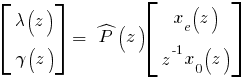(4)(5)

The polyphase matrix now performs the wavelet transform. If we setandto one and we makeandzero, i.e.is the unit matrix, then the wavelet transform is referred to as the lazy wavelet transform [Swe96a]. However, I would like to rename it to the femmelet transform (femmelette being French for wimp). The femmelet transform does nothing more than splitting the input signal into even and odd components. The polyphase matrix will be used later on to build a very flexible wavelet transform.

Now let’s move on to the right part of figure 1, the inverse wavelet transform. Here we have to deal with upsampling after which some filtering is performed. Upsampling is nothing more than inserting a zero in between every two samples and the consequence of this is that the filter will perform a lot of multiplications by zero, again a waste of computing time. Since the idea of moving the subsampling in front of the filters worked rather well for the forward wavelet transform, we will try a similar approach for the inverse wavelet transform, i.e. moving the upsampling behind the filters.

We look again at (2) but now imagine it as being the result of filtering an upsampled sequence of samples. If we assume that the inserted zeroes are the odd samples, then all the terms with an odd-numbered x(z) vanish and we can divide the output samples y(z) in odd and even sequences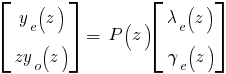(7)

where P(z) is a second polyphase matrix, the dual of the first,

Note that when we ignore the hats in (5), (8) is the transposed version of (5). This polyphase matrix performs the inverse wavelet transform. In case of the femmelet transform P(z) will be the unit matrix as well.

In figure 3 we have redrawn the filter stage of figure 1, this time using the polyphase matrices. The delays we had in (4) and (7) are incorporated in the split– and merge boxes. From this figure it will be clear that the condition for perfect reconstruction now can be written as(9)

Here we have time-reversed one of the two polyphase matrices because in order for (9) to hold we need to cancel the delays caused by the polyphase matrices (a FIR filter is a delay line, see figure 2).

If we assume that P(z) is invertible and if we use Cramer’s rule to calculate its inverse, we find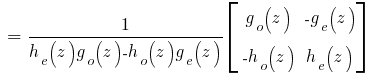(10)

From this it follows that if we demand that the determinant of P(z) = 1, i.e. he(z)go(z) – ho(z)ge(z) = 1, then not only will P(z) be invertible, but also(12)

In the special case that h =and g =the wavelet transform is orthogonal, otherwise it is biorthogonal.

If the polyphase matrix has a determinant of 1, then the filter pair (h,g) is called complementary. If the filter pair (h,g) is complementary, so is the filter pair ().

Note that if the determinant of P(z) = 1 the filters he(z) and ho(z) have to be relatively prime and we will exploit this property in the section on filter factoring. Of course the pairs ge(z) and go(z), he(z) and ge(z) and ho(z) and go(z) will also be relatively prime.

Summarizing we can state that the problem of finding an invertible wavelet transform using FIR filters amounts to finding a matrix P(z) with determinant 1. From this matrix the four filters needed in the invertible wavelet transform follow immediately. Compare this to the definition of the continuous wavelet transform at the beginning of the wavelet tutorial. We sure have come a long way! But there is more to come.

## 4. Intermezzo: Laurent polynomials

As an intermezzo some algebra will now be presented, which we will need in the following sections.

The z-transform of a FIR filter is given by(13)

This summation is also known as a Laurent polynomial or Laurent series. A Laurent polynomial differs from a normal polynomial in that it can have negative exponents. The degree of a Laurent polynomial h is defined as(14)

so that the length of the filter is equal to the degree of the associated polynomial plus one. Note that the Laurent polynomial zp has degree zero. The sum or difference of two Laurent polynomials is again a Laurent polynomial and the product of two Laurent polynomials of degree a and b is a Laurent polynomial of degree a+b. Exact division is in general not possible, but division with remainder is. This means that for any two Laurent polynomialsand, withthere will always exist a Laurent polynomialwith, and a Laurent polynomialwithso that

This division is not necessarily unique.

Finally we remark that a Laurent polynomial is invertible if and only if it is of degree zero, i.e. if it is a monomial.

In the following sections we will unleash the power of algebra on the polyphase matrices. The result will be an extremely powerful algorithm to build wavelet transforms.

## 5. Lifting

As will be clear from our intermezzo the polyphase matrix is a matrix of Laurent polynomials and since we demanded that its determinant be equal to 1, we know that the filter pair (h,g) is complementary. The lifting theorem [Dau97] now states that any other finite filter gnew complementary to h is of the form(16)

where s(z2) is a Laurent polynomial. This can be seen very easily if we write gnew in polyphase form (see also ) and assemble the new polyphase matrix as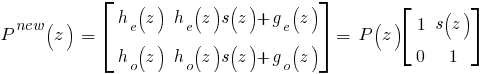(17)

Similarly, we can apply the lifting theorem to create the filternew(z) complementary to(z) (recall the identities from (11))(18)

with the new dual polyphase matrix given by

What we just did is called primal lifting, we lifted the low-pass subband with the help of the high-pass subband. figure 4 shows the effect of primal lifting graphically.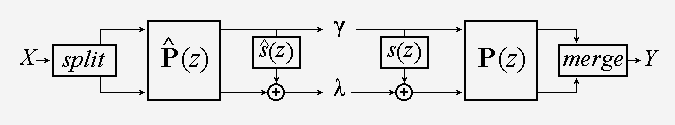Figure 4. Primal lifting, the low-pass subband is lifted with the help of the high-pass subband.

We can also go the other way, that is lifting the high-pass subband with the help of the low-pass subband and then it is called dual lifting. For dual lifting the equations become

and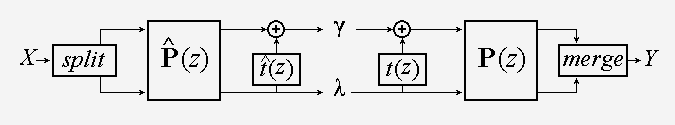Figure 5. Dual lifting, the high-pass subband is lifted with the help of the low-pass subband.

After all these equations and figures it should be clear how things work in the lifting scheme and we can explain why this technique is called lifting. If we start for example with the femmelet transform then both the polyphase matrices are simply equal to the unit matrix. When we apply a primal- and/or a dual lifting step to the femmelet transform we get a new wavelet transform which is a little more sophisticated. In other words, we have lifted the wavelet transform to a higher level of sophistication. We can perform as many lifting steps as we like and therefore we can build highly sophisticated wavelet transforms.

The inverse lifting transform now also begins to take shape. If we start with a femmelet transform we only split the input stream into an even and an odd stream. Then we lift one of these streams as in the left of figures 4 and 5 by applying a Laurent polynomial to the other and adding it to the first. We can very easily undo this lifting step by again applying the same Laurent polynomial to the other stream and then subtract it from the first. In other words, inverting a lifting transform is the same as changing all the signs of the lifting Laurent polynomials in figures 4 and 5 and run it backwards, i.e. start at the output. Inverting the lifting scheme this way will always work! From this we can conclude thatand.

From the figures 4 and 5 we can see another interesting property of lifting. Every time we apply a primal or dual lifting step we add something to one stream. All the samples in the stream are replaced by new samples and at any time we need only the current streams to update sample values. In other words, the whole transform can be done in-place, without the need for auxiliary memory. This is the same as with the fast Fourier transform, where the transformed data also takes the same place as the input data. This in-place property makes the lifting wavelet transform very attractive for use in embedded applications, where memory and board space are still expensive.

We conclude this section with a note on terminology. In lifting literature the dual lifting step is also referred to as the predict step, while the primal lifting step is also referred to as the update step. The idea behind this terminology is that lifting of the high-pass subband with the low-pass subband can be seen as prediction of the odd samples from the even samples. One assumes that, especially at the first steps, consecutive samples will be highly correlated so that it should be possible to predict the odd ones from the even ones (or the other way around). The update step, i.e. lifting the low-pass subband with the high-pass subband, then is done to keep some statistical properties of the input stream, usually at least the average, of the low-pass subband.

## 6. Factoring filters

In the previous section we lifted a wavelet transform to a more sophisticated level. Of course we can also do the opposite, i.e. we can factor the FIR filters of an existing wavelet transform into lifting steps. This would be very useful because a lot of research has already been performed on designing wavelet filters for all kinds of applications and factoring these filters will allow us to benefit easily from this research. So how do we go about?

Starting with for instance (22) we can go the other way by writing(24)

The form of (24) is identical to a long division with remainder of Laurent polynomials, whereis the remainder. If we rewrite (23) a little as well, we obtain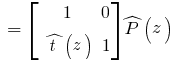(25)

and we see that for the polyphase matrix we have to perform two long divisions in order to extract one lifting step. Once we have extracted one such lifting step we can continue by extracting more lifting steps from the new polyphase matrix until we end up with the unit matrix, or a matrix with only two constants on its main diagonal. In fact, in [Dau97] it is proven that it will always be possible to do this when we start with a complementary filter pair (h,g), i.e. P(z) can always be factored into lifting steps:(26)

In (26) K1 and K2 are scaling constants unequal to zero. If scaling is not desired for some reason, it is even possible to factor the scaling matrix into four more lifting steps, one of which can be combined with the last real lifting step so that factoring a scaling matrix costs three extra lifting steps [Dau97].

To perform a long division with remainder on Laurent polynomials we can use the Euclidean algorithm for Laurent polynomials . The Euclidean algorithm was originally developed to find the greatest common divisor (gcd) of two natural numbers, but we can extend it to Laurent polynomials as well. In [Dau97] it is given as:

Take two Laurent polynomialsand, with. Letandand iterate the following steps, starting from i = 0(27)

Thenwhere n is the smallest number for which.

The `%’-symbol in the second line of (27) means mod, i.e. integer divide with remainder but only keeping the remainder, and it is the same symbol as used in the C programming language for the mod operation. The `/’-symbol in the third line is the C-language div operator. The result of this algorithm can be written as(28)

which looks very much like a series of lifting steps. The gcd found might not be unique since it is defined only up to a factor, i.e. there are several factorizations possible. This turns out to be an advantage because it allows us to select the factoring which best suits our needs.

## 7. Example

To summarize the theory of the previous sections we now present a detailed example.

Suppose we are given the following wavelet transform filters:(29)

which we want to use in a lifting scheme. What do we have to do?

The first step is to assemble the corresponding polyphase matrix. Because we have read all the footnotes we recall that the polyphase representation is given by

and apply this to the two analyzing filters to obtain

which means that

and thus

With the help of (11) we can now assemble the synthesizing polyphase matrix as well. First we check the determinant of (33):(34)

so we have to scale (11) a bit before using the equalities to get:(35)

Do not get confused here, P(z) is not time-reversed! Remember, if we want to check (9) we have to time-reverse one of the polyphase matrices. From (35) we can find the synthesizing filters as follows:(36)

thus

so that

Note that it is not necessary to actually calculate the synthesizing filters because of the simple reversibility of the forward transform. We have done it here just to illustrate how things work.(39)

which means that we have to solve the following two equations:

We use the Euclidean algorithm withandand perform one step. Now note that there are three possibilities for q1, and thus for b1, depending on which two terms of a0 you want to match with b0:

Note also that we have found three greatest common divisors. If we choose the middle line of (41) as the factorization we have a symmetrical one, which goes nicely withas well, and we arrive at the following decomposition:(42)(43)

This equation gives a fully factored version of the filters from (29). If we finally use (43) with (4) we can display our wavelet transform graphically as in figure 6 while the corresponding wavelet and scaling function are displayed in figure 7.

From figure 6 we can generalize the lifting steps as:(44)

to emphasize the in-place calculation property of the lifting transform.

## 8. Lifting properties

The lifting scheme has some properties which are not found in many other transforms. figure 6 shows a few of these properties and we will now discuss some of the most important.

The inverse transform is immediately clear: change the signs of all the scaling factors, replace "split" by "merge" and go from right to left, i.e. reverse the data flow. This easy invertibility is always true for the lifting scheme.

Lifting can be done in-place (44): we never need samples other than the output of the previous lifting step and therefore we can replace the old stream by the new stream at every summation point. Not immediately clear from this figure is that when we iterate a filter bank using in-place lifted filters we end up with interlaced coefficients. This can be seen as follows. We split the input in odd- and even-numbered samples and perform the in-place lifting steps. After one complete step the high-pass filtered samples, the wavelet coefficients, sit in the odd-numbered places and the low-pass filtered samples sit in the even-numbered places. Then we perform another transform step, but only using the low-pass filtered samples, so that this sequence will again be divided into odd- and even-numbered samples. Again the odd-numbered samples are transformed into wavelet coefficients, while the even-numbered samples will be processed further so that in the end all wavelet coefficients will be interlaced.

The third important property has not been mentioned yet, but it shows clearly from figure 6: lifting is not causal. Usually this is not really a problem, we can always delay the signal enough to make it causal, but it will never be real-time. In some cases however it is possible to design a causal lifting transform.

The last important property we will mention here is the calculation complexity. In [Dau97] it is proven that for long filters the lifting scheme cuts computation complexity in half, compared to the standard iterated FIR filter bank algorithm. This type of wavelet transform has already a complexity of N, in other words, much more efficient than the FFT with its complexity of Nlog(N) and lifting speeds things up with another factor of two. This is where the title of this tutorial comes from: it is a fast wavelet transform and therefore we will refer to it as the fast lifting wavelet transform of FLWT.

## 9. Integer lifting

The last stage of our voyage to the ultimate  wavelet transform is the stage where we make sure that the wavelet coefficients are integers. In classical transforms, including the non-lifted wavelet transforms, the wavelet coefficients are assumed to be floating point numbers. This is due to the filter coefficients used in the transform filters, which are usually floating point numbers. In the lifting scheme it is however rather easy to maintain integer data, although the dynamic range of the data might increase. That this is possible in the lifting scheme has to do with the easy invertibility property of lifting.

The basic lifting step is given in (44) and we rewrite it here a little modified as [Uyt97c]:(45)

Because the signal part y(z) is not changed by the lifting step, the result of the filter operation can be rounded, and we can write:

where we have used round() to denote the rounding operation. (46) is fully reversible:(47)

and this shows the most amazing feature of integer lifting: whatever rounding operation is used, the lifting operation will always be reversible.

As mentioned before the integer lifting transform can not guarantee the preservation of the dynamic range of the input signal. Usually the dynamic range doubles [Uyt97c], but there are schemes that can keep the dynamic range. In [Cha96] an interesting lifting transform with the so-called property of precision preservation (PPP) is described. This transform makes use of the two-complement representation of integers in a computer and the wrap-around overflows cause in this representation. The disadvantage of such a transform is that large coefficients may be represented by small values and it is therefore difficult to take decisions on coefficient values.

## 10. Coda

We have now finished our self-imposed task of transforming the CWT into a practical implementation. In this tutorial we have seen how we can use the lifting scheme to build a very versatile wavelet transform. After first optimizing the subsampled and upsampled FIR filters from the wavelet tutorial, through the use of some algebra we arrived at a scheme to build a wavelet transform using primal and dual lifting blocks. These modules allowed us to build any wavelet transform, which fits in the classical framework, and more. Adapting the lifting scheme we will be well armed: amongst our weaponry are such elements as  easy invertibility of any transform, in-place calculation of the transform and easy integer transform coefficients without losing any of its features. And there are many more features [Cal96], [Uyt97b], [Dau97].

However, this does not mean that this is the only way to go. There are probably as many wavelet transforms as there are wavelets. Due to the infinite variety of wavelets it is possible to design a transform which maximally exploits the properties of a specific wavelet , and of course this has been done. While researching wavelet theory I have stumbled upon morlets , coiflets, wavelants, slantlets, brushlets and wavelet packets to name a few. The lifting scheme on the other hand is a really general scheme, which makes it very suitable for experimenting while the in-place and integer properties make it extremely useful for embedded systems where memory is still expensive. With the application described in this report in mind, it will be clear that these are the reasons for studying the lifting scheme.

Finally, four remarks to conclude this tutorial:

• In [Uyt97b] the filter factoring algorithm is used to split the original filters in simpler filters and one primal and dual lifting step. These lifting steps are then used to make the original wavelet transform integer. This is some kind of hybrid (trans)form but very effective.

• Up to now we have only spoken about one-dimensional transforms. It is however easily possible to extend the lifting transform to the multi-dimensional case. Not only can the lifting transform be used in a classical separable multi-dimensional setting, but it can be made truly multi-dimensional. In [Uyt97a] the lifting transform is extended to a true two-dimensional transform, while in [Kov97] the complete theoretical foundations are laid out for any dimension. The principles behind lifting do not change at all in the multi-dimensional setting.

• One of the advantages of the lifting scheme as pointed out in for instance [Dau97] and [Cal96] is that the lifting scheme allows for an introduction into wavelet theory without the use of Fourier theory. We do not agree with this on the grounds that from the lifting scheme it is totally unclear why there should be wavelets in it at all. The concept of wavelets is completely unnecessary to understand the lifting scheme and therefore, we feel, it should not be used as an introduction to wavelet theory.

• The lifting scheme is constantly under development and is investigated by many. Recent additions are the lifting scheme in a redundant setting in order to improve the translation invariance [Sto98] and adaptive prediction schemes for integer lifting [Cla97].

## 11. Notes

 This follows easily once we know that the polyphase representation of a sequence of samples x(z) is given byThis equation has, by the way, an interesting property. If we express xe(z2) and xo(z2) in x(z) we arrive at the beautiful resulti.e. the polyphase representation can be seen as a digital equivalent of Euler’s formula.

 Like the Taylor series, Laurent series can be used to expand functions in.

 Why? We write the long division with remainder of a0 and a1 as a0 = q1 a1 + a2. But we can express a1 in a similar way as a1 = q2 a2 + a3 and a2 also, and so on. The row of remainders will eventually reach zero, a1 > a2 > … > an > an+1 = 0, and this is where it stops. The gcd of a0 and a1 is now an. However, we are more interested in the intermediate results a0 = q1( q2( … ( qnan + an+1) + …) + a3) + a2 (remember an+1 = 0) or written as in (28).

 Why? Becauseis the longest filter.

 That is, in our limited world, i.e. the context of this tutorial.

 "Our chief weapon is surprise. Surprise and fear. Fear and surprise. Our two weapons are fear and surprise. And ruthless efficiency. Our three weapons are fear, surprise, and ruthless efficiency. And an almost fanatical devotion to the Pope. Our four. No. Amongst our weapons. Amongst our weaponry… are such elements as fear, surprise."
From Monthy Python’s Flying Circus, series 2, episode 15, "The Spanish Inquisition" (1970).

 Compare this to the Fourier transform, where a sine will always be a sine.

 Very funny indeed, Morlet [Mor82] being more or less the inventer of wavelets, but used as such in [Wei94].

## 12. References

Books and papers

[Cal96] Calderbank, A. R. and I. Daubechies, W. Sweldens, B.-L. Yeo WAVELET TRANSFORMS THAT MAP INTEGERS TO INTEGERS. Proceedings of the IEEE Conference on Image Processing. Preprint, 1996. IEEE Press, 1997.

Internet resources

Besides traditional references (i.e. on paper) there are many Internet resources that deal with wavelets. Here I list a few that have proved to be useful. With these links probably every other wavelet related site can be found. Keep in mind however that this list was verified for the last time in October 2010.

The Wavelet Digest, a monthly electronic magazine currently edited by Wim Sweldens, is a platform for people working with wavelets. It contains announcements of conferences, abstracts of publications and preprints and questions and answers of readers. It can be found at and it is the site for wavelets.

Rice University, the home of Burrus [Bur98] et al, keeps a list of publications and makes available the Rice Wavelet Toolbox for MatLab.

The Katholieke Universiteit of Leuven, Belgium, is active on the net with wavelets, publications and the toolbox WAILI.

Amara Graps maintains a long list of links, publications and tools besides explaining wavelet theory in a nutshell.

There used to be a real lifting page, dedicated to Liftpack, a lifting toolbox. Unfortunately, it is no longer available but recently I found a copy on an old hard disk. Download it here.

Finally I would like to mention an interesting tutorial aimed at engineers by Robi Polikar from Iowa State University.

## 13. Fir2Lift – Automated Lifting Step Extraction

I wrote a program to automate the factoring of wavelet FIR filters into lifting steps. This program is written in ANSI-C and is available for downloading, sources included. Beware: it has the very first in user interfacing. Now with MSVC6 project files and clean UNIX makefile.